Image Processing Toolbox User's GuideDefining the Transformation Data

Before you can perform a spatial transformation, you must first define the parameters of the transformation. The following sections describe two ways you can define a spatial transformation.

With either method, you pass the result to the `maketform `function to create the `TFORM` structure required by `imtransform`.

Using a Transformation Matrix

The `maketform` function can accept transformation matrices of various sizes for N-D transformations. Because `imtransform` only performs 2-D transformations, you can only specify 3-by-3 transformation matrices.

For example, you can use a 3-by-3 matrix to specify any of the affine transformations. For affine transformations, the last column must contain 0 0 1 (`[zeros(N,1); 1]`). You can specify a 3-by-2 matrix. In this case, `imtransform` automatically adds this third column.

The following table lists the affine transformations you can perform with `imtransform` along with the transformation matrix used to define them. You can combine multiple affine transformations into a single matrix.

 Affine Transform Example Transformation Matrix Translation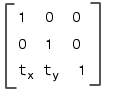`t`x specifies the displacement along the x axis `t`y specifies the displacement along the y axis. Scale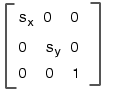`s`x specifies the scale factor along the x axis`s`y specifies the scale factor along the y axis. Shear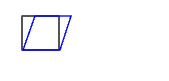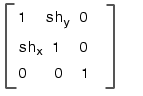`sh`x specifies the shear factor along the x axis`sh`y specifies the shear factor along the y axis. Rotation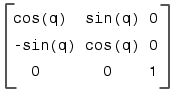`q` specifies the angle of rotation.

Using Sets of Points

Instead of specifying a transformation matrix, you optionally use sets of points to specify a transformation and let `maketform` infer the transformation matrix.

To do this for an affine transformation, you must pick three non-collinear points in the input image and in the output image. (The points form a triangle.) For a projective transformation, you must pick four points. (The points form a quadrilateral.)

This example picks three points in the input image and three points in the output image created by the translation performed in Example: Performing a Translation. The example passes these points to `maketform` and lets `maketform` infer the transformation matrix. The three points mark three corners of one of the checkerboard squares in the input image and the same square in the output image.

• ```in_points = [11 11;21 11; 21 21]

out_points = [51 51;61 51;61 61]

tform2 = maketform('affine',inpts,outpts)
```

To view the transformation matrix created by `maketform`, look at the `tdata` field of the `TFORM` structure. The following shows the transformation matrix for the forward transformation that is stored in the `T` field of the `tdata` structure. Note that `maketform` created the same as transformation matrix as the one created in Step 2: Define the Spatial Transformation. For more information about the `TFORM` structure, see Contents of a TFORM Structure.

• ```tform2.tdata.T

ans =

1     0     0
0     1     0
40    40     1
```Example: Performing a Translation Creating TFORM Structures